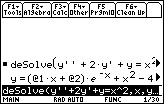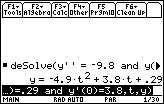# Knowledge Base

## Solution 17214: Solving Second Order Differential Equations on the TI-89 Family, TI-92 Plus and Voyage™ 200 Graphing Calculator.

### How do you solve a second order differential equations on a TI-89 Family, TI-92 Plus and Voyage 200 Graphing Calculator?

First and second order differential equations can be solved by using the deSolve( command.

1. First Order Example

Solver the equation y''+2y'+y=x^2.

Solution: Start by clearing the calculator's home screen and following the instructions below to solve using the deSolve( command.

• Press [CATALOG] and scroll to deSolve( or choose deSolve( from the (F3) 'Calc' menu.
• Enter y''+2y'+y=x^2, x, y)  Note: To get ', press the [2ND] [ ].
• Press [ENTER] to display the answer. The variables @1, @2 are constants.2. Second Order Example:
A ball is tossed straight up from an initial height of 0.29 meters and with an initial velocity of 3.8m/s. Solve the second order differential equation below to model the height of the ball over time:

y"=-9.8, y(0)=.29, y'(0)=3.8

Solution: Start by clearing the calculator's home screen and following the instructions below to solve using the deSolve( command.

• Press [CATALOG] and scroll to deSolve( or choose deSolve( from the (F3) 'Calc' menu.
• Press [Y] then [2ND] [ ] [2ND] [ ' ] [=].
• Enter [(-)] 9.28 [CATALOG].
• Choose 'and' from the list and press [ENTER].
• Input the remaining equations separated by 'and'.
• After inputting the final equation type [ , ] [T] [ , ] [Y] [ ) ].